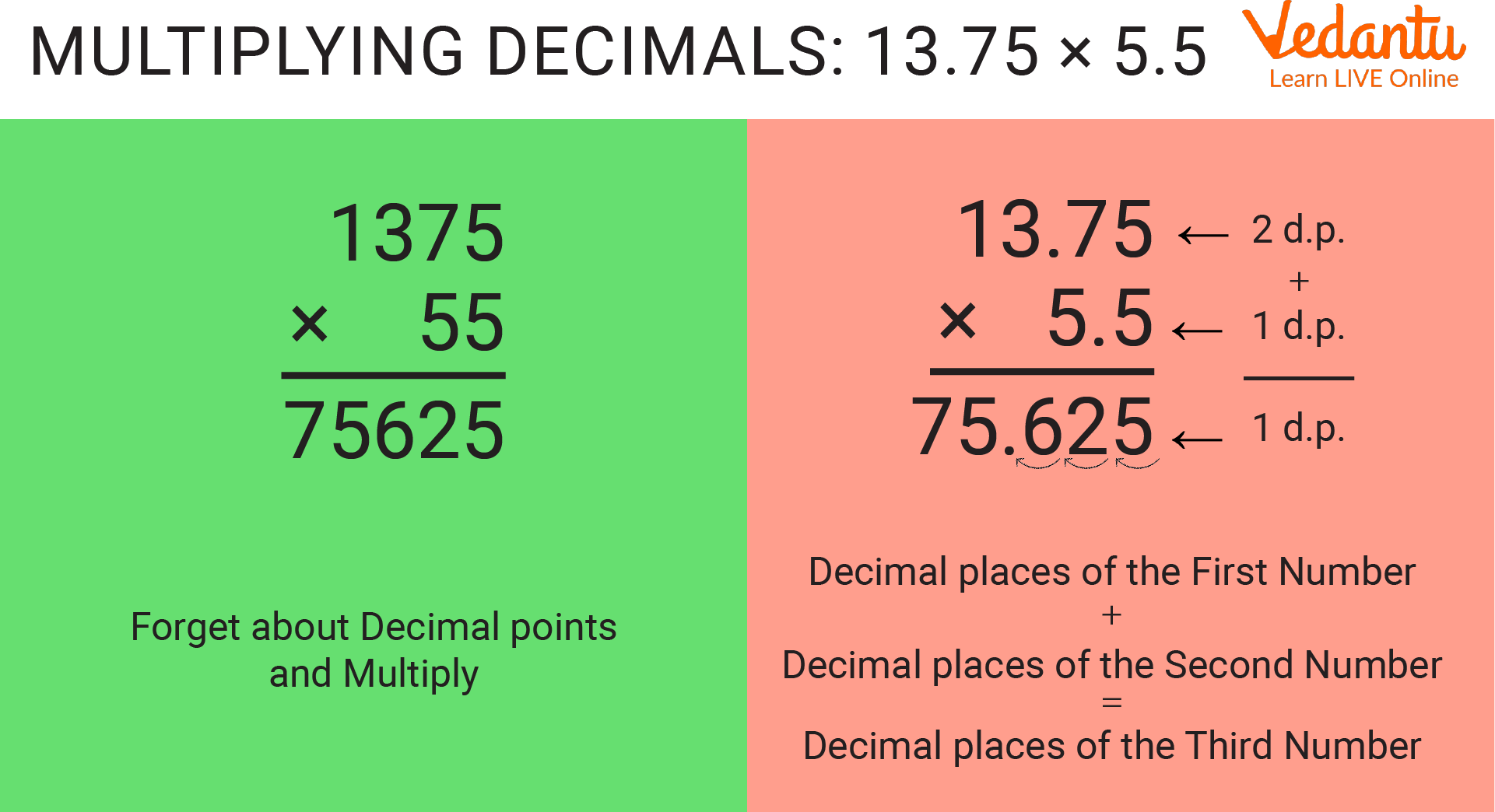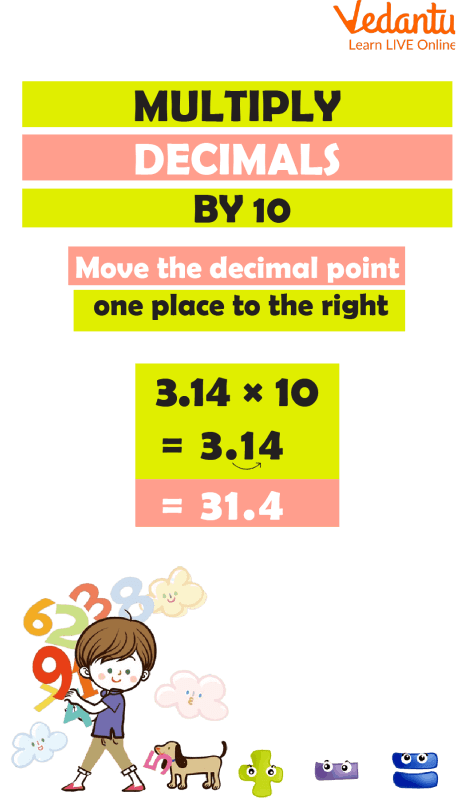Courses
Courses for Kids
Free study material
Free LIVE classes
More

# Decimal Multiplication for KidsLIVE
Join Vedantu’s FREE Mastercalss

## What is Decimal Multiplication? An Introduction

The placement of the decimal point differs from how you multiply whole numbers when dividing decimals. A decimal is multiplied by a whole number exactly like a whole number, but the decimal point is added. Multiplying decimals results in a decimal point. This result has a total of ten decimal places. The placement of decimal points differs from multiplying whole numbers. Keep reading to know more about decimal multiplication.Multiplying Decimals

## How Do Decimals Work?

A fractional point (also called a 'decimal separator') separates the whole part from the fractional part of a number with a point or a dot. However, in the UK, children are taught to write a point as the decimal separator, as opposed to a comma. To help children understand decimal numbers, the connection between decimal numbers and fractions will be discussed in the next section.

## Calculating Decimal Multiplication Using Whole Numbers

The only difference between multiplying decimals with whole numbers and multiplying them with decimals is where the decimal point is placed. You can perform this operation by following these steps:

• Step 1: Multiply the two numbers without the decimal point at first.

• Step 2: Once you have multiplied the fractional number, count how many decimal places there are. The product will have the same number of decimal places when you divide two numbers.

• Step 3: Add the decimal point to the result obtained in Step 2.

For example,

$6.7281 \times 10 =67.281$

$6.7281 \times 100 =672.81$

$6 .7281 \times 1000 =6728.1$

This applies when you procreate by multiples of 10.

For sample:

50 × 2.5 = 5 × 10 × 2.5 = 5 × 25 = 125

## Multiplying Two Decimal Numbers

Multiplying two-digit decimal numbers will be explained in this section. We have to sum up the decimal places in both the whole number and the product, which must equal the sum of the decimal places in each given number.

The following are the steps for multiplying two decimal numbers:

• Step 1: Multiply the two numbers normally without the decimal point.

• Step 2: After multiplying, see how many decimal places there are in both numbers. This is the number of decimal places in the product obtained after dividing two numbers.

• Step 3: Add a decimal point to the result based on Step 2.

Let’s check an example:

Step 1: Multiply the two figures as two whole calculations.

36 × 13 = 468.

Step 2: Currently, there's one number each after the decimal point in the factors.

So, 3.6 × 1.3 = 4.68.

Factor 3.6 can be compared to 4 and 1.3 to 1. Thus, their produce can live valued to be 4. This will help you in certifying the placement of the decimal point.

## The Multiplication of Decimals by Ten, One Hundred, and One Thousand

A decimal multiplied by 10, 100, 1000, or any other power of 10 simply shifts the decimal point to the right in as many places as the number of zeros.

• As the number 10 has 1 zero, the decimal point shifts one place towards the right when we multiply by 10.

• The decimal point shifts two places to the right when we multiply a decimal by 100.

• In the same way, a decimal point shifts three places to the right after getting multiplied by 1000.

The following example, 2.32 × 10 = 23.2, 2.32 × 100 = 232, 2.32 × 1000 = 2320.Multiplying Decimals by 10

Calculate the value of 4.469 by multiplying it by 10. Then multiply 4.469 by 100. The final step is to multiply 4.469 by 1,000.

$4.469 \times 10 = 44.690$

$4.469 \times 100 = 446.900$

$4.469 \times 1000 = 4469.000$

## How to Multiply Decimal Numbers?

To multiply the decimals together, arrange them in a line. Ignore the decimal and multiply the decimals as if they were conventional numbers. The decimal places in each of the two numbers are counted. How many times you move the decimal in your solution depends on the number of decimal places in the decimal.

## Summary

Decimals are needed for multiplying and dividing. If you have worked with whole numbers, you will work with decimals as well, but you will need to understand where the decimal point is. As a result of multiplying decimals, the product contains the same number of decimal places as the factors. We hope you found sufficient help with this article and we were able to resolve your queries, you can visit our website to try out Maths worksheets to enhance your Mathematics skills.

Last updated date: 28th Sep 2023
Total views: 116.1k
Views today: 2.16k

## FAQs on Decimal Multiplication for Kids

1. How do you multiply decimals according to the rule of multiplication?

The multiplication rules for decimals are as follows:

• Consider whole numbers to be whole numbers when multiplying.

• A product can have more decimal places than its digits by inserting zeros before the decimal point. This will ensure that only one zero appears to the left of the decimal point.

• If a product has the decimal point in such a way as to have the same number of decimal places as all the multiplicands and multipliers, then the product should have the same number of decimal places.

• There is no need to add trailing zeros in the fractional part of the resultant product.

2. How do you multiply numbers lower than 1?

To reproduce two numbers lower than 1, follow the way given below.

Step 1: First, ignore the decimal point and multiply the two figures typically.

Step 2: After the increase, number the total number of decimal places in multiplicand and the multiplier. The product procured after addition will retain this total number of decimal points.

Step 3: Place the decimal point in the obtained product following Step2.

For illustration 0.2 × 0.4 = 0.08.

3. What is the formula for multiplying a decimal by a whole number?

Performing regular multiplication without considering the decimal point at the beginning is similar to multiplying a decimal by a whole number. After analysing the numbers, the decimal point is placed according to their decimal places. Therefore, all multiplicands and multipliers in the product must be placed in a way that makes the decimal point equal to the sum of the decimal places in the product.